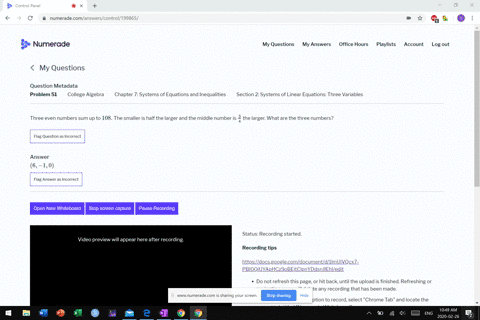Sign up for our free STEM online summer camps starting June 1st!View Summer Courses### Three even numbers sum up to $108 .$ The smaller …

03:28
MB

Need more help? Fill out this quick form to get professional live tutoring.

Get live tutoring
Problem 50

For the following exercises, solve the system for $x, y,$ and $z$
\begin{aligned} \frac{x-1}{3}+\frac{y+3}{4}+\frac{z+2}{6} &=1 \\ 4 x+3 y-2 z &=11 \\ 0.02 x+0.015 y-0.01 z &=0.065 \end{aligned}

$\left(-\frac{54}{17}, \frac{377}{17}, \frac{191}{17}\right)$

## Discussion

You must be signed in to discuss.

## Video Transcript

all right. Questions. 50. They're trying to put all this stuff in together right here. Okay, so, um, basically, Well, we're gonna clear out these problems of fractions, and each one has its own little trick. The 2nd 1 was sorry. That so. This one here. All right. We want to multiply by number. That 34 and six. Well, over into evenly animals by this one. About 12. Guess that guess before X minus one. Close three explosive Y plus three plus two Z plus two because 12. All right. Um, this one's good the way it is right now. Um, this last one. Uh well, let's Let's just go ahead and simplify this. This is or X minus four. So I don't need this room under you. Plus three. Why? Plus nine plus two z plus or he was 12. So that becomes or ex plus three. Why? Plus two z equals What? Yeah. 12 clothes for 16. One is for 12 of this nine story. Right? So re close to that second equation Arabic. Not the same. Hey, this one right here. The last one smoked by this one by 1000. So move all the decimal places over three places. This becomes 20 X was 15. Why? Minus, um, Tensy equal 65. Bring down the other equations, which I have already. So the second equation there was for eggs close to me. Why Miners, Susie People's 11. That requires here that I have Where? Plus three. Why? Plus two z equals three. All right, So these two equations here, I can subtract them right now, and I will get you see 00 and Weiss attract up like it or Z equals three. Minus 11 is negative. Eight. So Z equals name, too. Okay, then I want Thio One memo by this equation by five Moved by this equation by five. That will allow me to combine it with the one above it. So this becomes 20 x us with teen. Why? Wow. Five minus 10. Do you want miners? Tensy People's 55. Okay, Well, um, Z is definitely negative too. But X and y can be determined because, uh, these two planes, when I subtract these two, I get zero equals 10. Right? And that is never troops as a false statement. So these two planes in parallel, um, does cross this plane and what we know is that crosses that. See, it's negative, too. There is not a unique solution to this system.